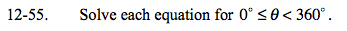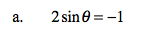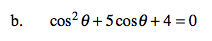### Home > A2C > Chapter 12 > Lesson 12.1.3 > Problem12-55

12-55.$\text{sin}\theta=-\frac{1}{2}$

Now use what you remember about the unit circle to solve for θ. Remember that there may be more than one solution.Treat cosθ as you would x and factor. Now set the factors equal to zero. Note that instead of having x = something, you will have cosθ = something.

If cosθ = −1, θ = ?